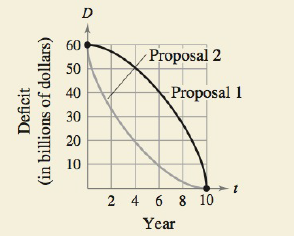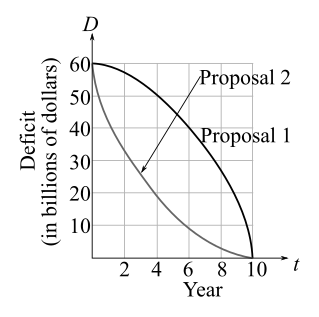Chapter 5.5, Problem 54E### Calculus: An Applied Approach (Min...

10th Edition
Ron Larson
ISBN: 9781305860919

#### Solutions

Chapter
Section### Calculus: An Applied Approach (Min...

10th Edition
Ron Larson
ISBN: 9781305860919
Textbook Problem
5 views

# HOW DO YOU SEE IT? A state legislature is debating two proposals for eliminating the annual budget deficits after 10 years. The rate of decrease of the deficits for each proposal is shown in the figure.(a) What does the area between the two graphs represent?(b) From the viewpoint of minimizing the cumulative state deficit, which is the better proposal? Explain your reasoning.

(a)

To determine

What does the area between the two graphs represent in the following figureExplanation

Given Information:

The rate of decrease of annual budget deficits by two different proposals for 10 years is shown in the following graph:

A state legislature is debating two proposals for eliminating the annual budget deficits after 10 years. The rate of decrease of deficits for each proposal is shown in the given graph...

(b)

To determine

Which of the two proposals is the better proposal from the viewpoint of minimizing the cumulative state deficit?

### Still sussing out bartleby?

Check out a sample textbook solution.

See a sample solution

#### The Solution to Your Study Problems

Bartleby provides explanations to thousands of textbook problems written by our experts, many with advanced degrees!

Get Started

#### In Exercises 21-24, find the distance between the given points. 21. (1, 3) and (4, 7)

Applied Calculus for the Managerial, Life, and Social Sciences: A Brief Approach

#### In problems 9 and 10, which of and are subsets of? 10.

Mathematical Applications for the Management, Life, and Social Sciences

#### cosh 0 = a) 0 b) 1 c) e22 d) is not defined

Study Guide for Stewart's Single Variable Calculus: Early Transcendentals, 8th

#### What is the general purpose of a manipulation check?

Research Methods for the Behavioral Sciences (MindTap Course List)